# High Energy Physics Theory Network

A collaboration and citation network on physics theory

The High energy physics theory network is a collaboration and citation network including a subset of papers from the High Energy Physics Theory section of the arXiv.

## Examples

### Basic Examples

Retrieve the graph:

 In:=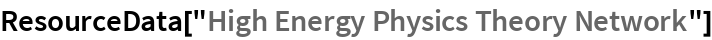Out=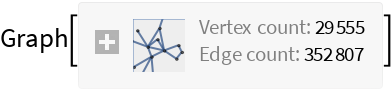Summary properties:

 In:=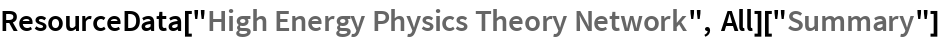Out=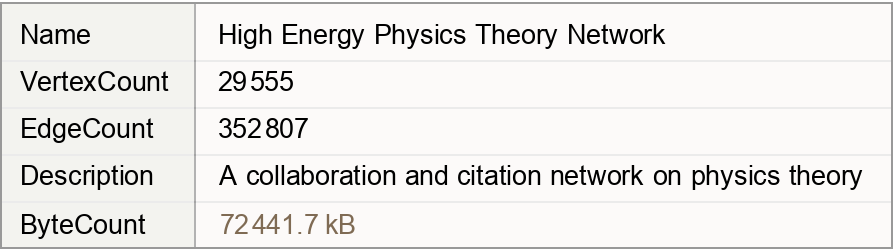### Basic Applications

Pick the author with the most collaborations:

 In:=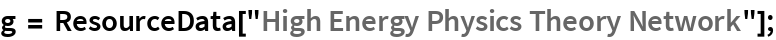In:=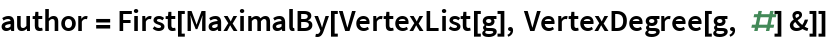Out=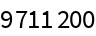Visualize the network around the author with the most collaborations:

 In:=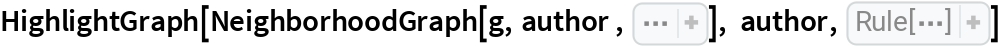Out=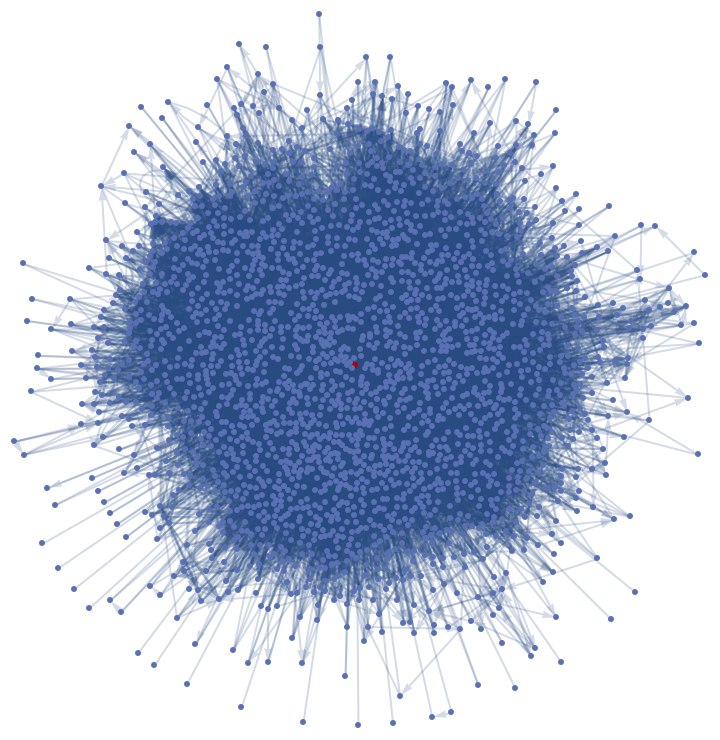Wolfram Research, "High Energy Physics Theory Network" from the Wolfram Data Repository (2019)